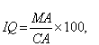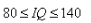JEE  >  Test: Linear Inequalities In One Variable And Two Variables

# Test: Linear Inequalities In One Variable And Two Variables

Test Description

## 10 Questions MCQ Test Mathematics (Maths) Class 11 | Test: Linear Inequalities In One Variable And Two Variables

Test: Linear Inequalities In One Variable And Two Variables for JEE 2023 is part of Mathematics (Maths) Class 11 preparation. The Test: Linear Inequalities In One Variable And Two Variables questions and answers have been prepared according to the JEE exam syllabus.The Test: Linear Inequalities In One Variable And Two Variables MCQs are made for JEE 2023 Exam. Find important definitions, questions, notes, meanings, examples, exercises, MCQs and online tests for Test: Linear Inequalities In One Variable And Two Variables below.
Solutions of Test: Linear Inequalities In One Variable And Two Variables questions in English are available as part of our Mathematics (Maths) Class 11 for JEE & Test: Linear Inequalities In One Variable And Two Variables solutions in Hindi for Mathematics (Maths) Class 11 course. Download more important topics, notes, lectures and mock test series for JEE Exam by signing up for free. Attempt Test: Linear Inequalities In One Variable And Two Variables | 10 questions in 10 minutes | Mock test for JEE preparation | Free important questions MCQ to study Mathematics (Maths) Class 11 for JEE Exam | Download free PDF with solutions
 1 Crore+ students have signed up on EduRev. Have you?
Test: Linear Inequalities In One Variable And Two Variables - Question 1

### What is the region represented by x > 0 and y < 0?

Detailed Solution for Test: Linear Inequalities In One Variable And Two Variables - Question 1

If x >0 then x will be positive and y less than 0 means it gets negative value
that means it lies in 4th quadrant.

Test: Linear Inequalities In One Variable And Two Variables - Question 2

### By solving inequality 3(a - 6) < 4 + a, answer will be

Detailed Solution for Test: Linear Inequalities In One Variable And Two Variables - Question 2

Numbers obtained in three throws are 5,3 and 6
Let the number obtained in fourth throw be x
Now, Sum > 20
5 + 3 + 6 + x > 20
14 + x > 20
x > 6(never wins)

Test: Linear Inequalities In One Variable And Two Variables - Question 3

### Find the solution for the pair of inequations x > 1 and x < -1

Detailed Solution for Test: Linear Inequalities In One Variable And Two Variables - Question 3

x > 1 means x is greater than 1 in this equation the values of x can be 2,3,4,5.............∞
x < -1 means is less than -1 therefore values of x can be -2, -3, -4, -5............ -∞
So no common point for x
Hence there will be no solution

Test: Linear Inequalities In One Variable And Two Variables - Question 4

The solution of the inequality 3(2-x)≥2(1-x) for real x is :

Detailed Solution for Test: Linear Inequalities In One Variable And Two Variables - Question 4

3(2 - x) ≥ 2(1 - x)
6 - 3x ≥ 2 - 2x
4 ≥ x

Test: Linear Inequalities In One Variable And Two Variables - Question 5

Find the solution for the pair of solution x > 1 and x > -1

Detailed Solution for Test: Linear Inequalities In One Variable And Two Variables - Question 5

x > 1 and x > -1
Take two points 1,-1 on number line
Therefore x > 1

Test: Linear Inequalities In One Variable And Two Variables - Question 6

IQ of a person is given by the formulawhere MA is mental age and CA is the chronological age. Iffor a group of 12 years old children, find the range of their mental age.

Detailed Solution for Test: Linear Inequalities In One Variable And Two Variables - Question 6

IQ = (MA*100)/CA
and 80 ≤ IQ ≤ 140
80 ≤ (MA*100)/CA ≤ 140
80 ≤ (MA*100)/12 ≤ 140
960 ≤ (MA*100) ≤ 1680
9.6 ≤ MA ≤ 16.8

Test: Linear Inequalities In One Variable And Two Variables - Question 7

The graphical representation of x > 2 on number line is

Detailed Solution for Test: Linear Inequalities In One Variable And Two Variables - Question 7

x>2, the value of x is greater than 2. the value goes till infinity.
if X is equal or greater than 2 then we use solid dot, but it is only greater than 2
so the option c is correct.

Test: Linear Inequalities In One Variable And Two Variables - Question 8

The graphical representation of x ≤ -3 and x ≥ 3 on number line is

Test: Linear Inequalities In One Variable And Two Variables - Question 9

In a game a person wins a TV if in four throws of a dice he get sum greater than 20 .In three throws he got numbers as 5,3,6. What should be his fourth throw so that he wins a TV?

Detailed Solution for Test: Linear Inequalities In One Variable And Two Variables - Question 9

Numbers obtained in three throws are 3, 5 and 6.
Let the number obtained in fourth throw be x.
Now, Sum > 20
=> 5 + 3 + 6 + x > 20
=> 14 + x > 20
=> x > 20 – 14
=> x > 6 (no one wins)

Test: Linear Inequalities In One Variable And Two Variables - Question 10

The graphical solution of -1 < x < 2 on number line is

## Mathematics (Maths) Class 11

156 videos|176 docs|132 tests
 Use Code STAYHOME200 and get INR 200 additional OFF Use Coupon Code
Information about Test: Linear Inequalities In One Variable And Two Variables Page
In this test you can find the Exam questions for Test: Linear Inequalities In One Variable And Two Variables solved & explained in the simplest way possible. Besides giving Questions and answers for Test: Linear Inequalities In One Variable And Two Variables, EduRev gives you an ample number of Online tests for practice

## Mathematics (Maths) Class 11

156 videos|176 docs|132 tests